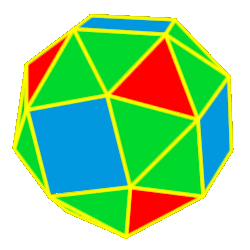# Polyhedra Snub Cuboctahedron

 The Snub CuboctahedronThe snub cuboctahedron  is created by either truncating (cutting off) tips of the cube or the octahedron. 38 total faces: 32 equilateral triangles and 6 squares 24 vertices: 4 triangles and 1 square 60 edges Dihedral angles:142 degrees, 59 minutes for the sqr-tri angle and 153 degrees, 14 minutes for the tri-tri angle